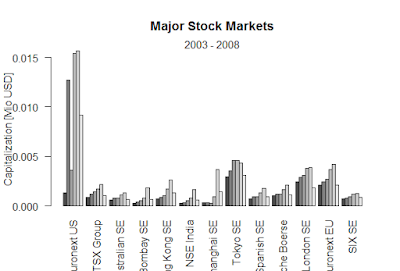Showing posts with label useful functions in R language. Show all posts
Showing posts with label useful functions in R language. Show all posts

## There are many useful functions in R language

rep(x,n) means repeat x times
p<- rep(1:4,2)
> p
 1 2 3 4 1 2 3 4

### Sequence function,

> seq(from, to, by)
indices <- seq(1,10,2)
> indices is c(1, 3, 5, 7, 9)

Length Function
> length(x)
x <- c(2, 5, 6, 9)
> length(x) returns 4

#### Divide continuous variable x into factor with n levels

cut(x, n)
 (1.99,2.7] (4.8,5.5]  (5.5,6.2]
 (8.3,9.01]
10 Levels: (1.99,2.7] ... (8.3,9.01]

### Black-Scholes formula-R

Black-Scholes formula-R > BlackScholes <- function(TypeFlag = c("c", "p"), S, X, Time, r, b, sigma) { TypeFla...